Difference schemes, theory of

The branch of numerical mathematics studying methods for finding approximate solutions to differential equations by replacing them by finite-difference equations (difference schemes).

The theory of difference schemes studies methods for constructing difference schemes, investigates the correctness of difference problems and the convergence of the solution of a difference problem to the solution of the original differential problem, and deals with the justification of algorithms for the solution of difference problems. A finite-difference method (also called a grid method) is a universal computing method enabling one to effectively solve complicated problems in mathematical physics, including non-linear problems. A distinctive feature of the contemporary theory of difference schemes is the orientation towards the construction and investigation of methods suitable for a computer.

Basic concepts.

A finite-difference method is applied in the theory of differential equations as an effective means for proving existence theorems. The problems in the theory of difference schemes are essentially quite different when the aim is mathematical computation. When finding an approximate solution to some problem in mathematical physics it is assumed in advance that this problem is well-posed; furthermore, the basic aim of the theory of difference schemes becomes that of finding and justifying the best method for solving the original differential equation, and of formulating general principles for constructing difference schemes with given properties for a wide class of problems in mathematical physics.

Below the basic concepts with which the theory of difference schemes operates — the concepts of approximation, stability and convergence — are presented for a fairly general example and one possible approach to the construction of a theory of difference schemes is illustrated.

Suppose one has to find the solution, in an-dimensional regionwith boundary, of a differential equation(1)

with additional (boundary, initial) conditions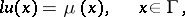(2)

whereandare linear differential operators and,are given functions. Let the problem (1), (2) be well-posed in a certain class of functions (that is, its solutionexists and depends uniquely and continuously on the input dataand). In a finite-difference method the region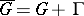is replaced by a discrete set of points — the grid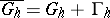. The parameter, the step of the grid, characterizes the density of the grid and usually, as, the sequence of grids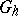tends to fill the whole region. The derivatives entering into (1), (2) are approximated on the grid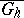by the corresponding difference relations. As a result a system of linear algebraic equations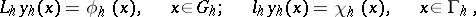(3)

is obtained, whereis the unknown grid function,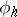,are given grid functions and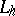,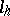are difference operators. The family of equations (3), which depends on the parameter, is called a difference scheme. Although equation (3) is obtained by approximating the original problem (1), (2), it can be considered as an independent mathematical object. The problem (1), (2) being well-posed does not imply, generally speaking, that the difference problem (3) is a well-posed (correct). Therefore, investigating the well-posedness of problem (3) is a first problem in the theory of difference schemes. In addition one studies, in the theory of difference schemes, whether the solutionof the difference problem converges, as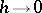, to the solutionof the original differential problem. Well-posedness and convergence are closely connected.

Let the set of grid functions onform the vector spaceand let the operatorsandact on this space. Introduce the norms,,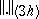in the spaces of solutions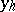and right-hand sides,.

The difference problem (3) is said to be well-posed (correct) if for all sufficiently small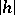and for anya unique solution exists and if the estimate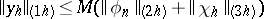(4)

is satisfied for some constantnot depending on. The latter property is called stability of the difference scheme and indicates that the solution depends uniformly, with respect to, on the input data. Estimates on the solution of the difference problem in terms of known right-hand sides of type (4) are called a priori estimates. Obtaining a priori estimates is at the basis of the theory of difference schemes.

To estimate the error, the solution of problem (3) is presented as a sumwhere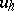is the projection of the solutionof the problem (1), (2) onto the space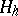and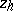is the error of the approximate solution. Since problem (3) is linear, one obtains for the error:(5)

where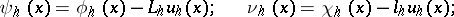the functionsandare called the errors of approximation, or local discretization errors, of the difference scheme (3) for equation (1) and the additional conditions (2), respectively. It is said that the scheme (3) is an-th order approximation to the problem (1), (2) if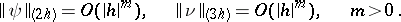The scheme (3) has-th order accuracy, or converges at the rate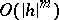, if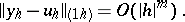Only one approximation is insufficient, generally speaking, for the convergence of the difference scheme: it is necessary to require further that the difference problem (3) be well-posed. Namely, the following assertion is true: If the difference scheme (3) is well-posed and has-th order approximation, then it converges at the rate(see ).

Other approaches to the construction of a theory of difference schemes are possible. Thus, in Lax's theory (see ) convergence of difference schemes is studied not in spaces of grid functions but in the solution space of the original differential problem; here the so-called equivalence theorem is proved: If the original problem (1), (2) is well-posed and the scheme (3) approximates problem (1), (2), then stability is necessary and sufficient for convergence. Other versions of the connection between stability and convergence are possible (see, for example, ). There has been some research also into the convergence of difference schemes in generalized solution spaces (see ).

Requirements for difference schemes.

For calculations on a modern computer it is not always sufficient to require that a difference scheme be convergent as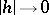. To be able to use an actual grid with a finite grid step requires the existence of a number of additional properties of the scheme. They amount to the fact that the difference scheme, besides having the usual approximation and stability properties, should also be a good model of the characteristic properties of the original differential equation. In addition, the difference scheme should satisfy definite conditions so that the computing algorithms are sufficiently simple in practice. Some of these additional requirements are considered below.

A uniform difference scheme (see , ) is understood to be a difference scheme of a type which does not depend either on the choice of the practical problem from a given class or on the choice of the difference grid. At all nodes of the grid for any problem from the given class the difference equations have the same form. Schemes related to uniform difference schemes are, in particular, transparent computation schemes for solving equations with rapidly changing or discontinuous coefficients. In transparent computation schemes the points of discontinuity in the coefficients do not have to be specified explicitly and the computation is carried out using the same formulas. Transparent computation schemes are widely used for calculating difference solutions to the equations in gas dynamics (see , , ).

A difference scheme may be conservative; this means that the given difference scheme has the same conservation law on the grid as the original differential equation. In particular, ifis a self-adjoint operator and the scheme (3) is conservative, thenis self-adjoint in, that is, a conservative scheme preserves the property of being self-adjoint.

The usual method for obtaining conservative uniform schemes is the so-called balance method or the integro-interpolation method. The essence of the balance method consists in approximating, on the difference grid, the integral conservation law (the balance equation) corresponding to the given differential equation. The balance method has found wide application in approximating equations with variable, including discontinuous, coefficients. Another group of methods for constructing differences schemes which preserve the property of self-adjointness and positive definiteness of the original operator are methods based on variational principles (Ritz' method, a finite-element method, see Difference scheme, variational; Ritz method).

In constructing difference schemes for the equations in gas dynamics the principle of being totally conservative has been applied (see ). For equations of hyperbolic type investigating the dispersion properties of the corresponding difference equations has turned out to be useful (see ).

If it is known that, as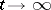, the solution of the original differential problem tends to zero, then it is natural to require the same for the solution of the approximating difference problem. Schemes with this property are said to be asymptotically stable (see ).

Another approach to constructing difference schemes of better quality is to try to obtain schemes which satisfy the same a priori estimates which are characteristic (and which cannot be improved) for the original differential equations (see ).

Economic difference schemes for multi-dimensional problems in mathematical physics.

In solving systems of difference equations which approximate non-stationary multi-dimensional problems in mathematical physics (with two or more space coordinates) specific difficulties arise connected with the fact that the number of arithmetic operations necessary to determine solutions at the next time layer increases sharply on refining the grid. At the same time the solution of one-dimensional non-stationary problems can be effectively treated by a marching technique, which is economic in the sense that it requires a finite (independent of the grid step) number of actions at a single point of the grid. In general, a difference scheme is said to be economic if the ratio of the number of actions necessary to find the solution at the next time layer to the number of nodes of the space grid does not depend on the number of nodes in the grid. The usual implicit difference schemes are not economic. A most effective method for constructing economic difference schemes is to reduce a multi-dimensional problem to a few one-dimensional problems (the alternating-direction method, the decomposition method) (see , , ).

New algorithms have also stimulated a new approach to the basic concepts in the theory of difference schemes: approximation, stability and convergence. For example, the concept of a total approximation or an approximation in the weak sense has turned out to be fruitful (see , ). An additivity principle has been formulated which enables one to construct, in the general case, economic difference schemes which have the property of total approximation (see ). The schemes with equations on graphs and vector schemes are further generalizations of alternating-direction difference schemes (see ).

Methods for investigating well-posedness and convergence of difference schemes.

For linear problems stability and consistency imply convergence. Therefore, the theory of difference schemes has concentrated mainly on obtaining a priori estimates, which imply well-posedness of the problems in some norm. Methods for obtaining a priori estimates for difference schemes are to a large extent analogous to the same methods in the theory of differential equations. For example, one can cite the following methods: separation of variables, Fourier transformation, maximum principle, the energy inequality.

Methods for solving grid equations.

Any grid method for differential equations results in large systems of linear algebraic equations. For example, for multi-dimensional problems the number of equations typically reaches the order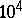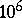. One-dimensional difference problems are usually solved by the shooting method (see ), which is a variation of the method of successive elimination of unknowns. Iteration methods are the most common methods for solving multi-dimensional grid equations. Iteration methods that are widely used in computing practice are the Richardson method with Chebyshev parameters; alternating-direction iteration methods; two-step iteration methods, which are a combination of the alternating-direction methods (internal iteration) and some classical method (external iteration). The method of upper relaxation and the alternate-triangular iteration method are also often used (see , ). The theory of iteration methods can be presented as a branch of the general theory of stability of difference schemes (see ).

There is a noticeable tendency to use direct (non-iterative) methods to solve multi-dimensional difference problems. Such methods are matrix shooting methods, discrete fast-Fourier transform methods and their generalizations, and the method of total representations.

Non-linear problems.

A theory of uniform difference schemes for non-linear equations of parabolic type has been developed (see ). Lax's theorem on the relation between stability and convergence has been generalized to the non-linear case (see , ). Difference schemes for non-linear elliptic equations (see , ) and for non-linear parabolic equations (see , ) have been considered. There are a number of general theorems on the well-posedness of difference schemes for the non-linear abstract Cauchy problem (see ). The convergence of difference schemes for the non-linear evolution equations has been studied (see , ).